# Python Program to Concatenate Two Strings

In this article, we've created some programs in Python, to concatenate string entered by user at run-time. Here are the list of programs:

• Concatenate String using + Operator
• Using list
• Using join()

For example, if two given strings are codes and cracker. Then after concatenating second string into first. The first string codes becomes codescracker after concatenation.

## Concatenate Two Strings using +

To concatenate two strings in Python, you have to ask from user to enter the first and second string, then concatenate using + operator just like addition as shown in the program given below. The question is, write a Python program to concatenate one string to other. Here is its answer:

```print("Enter the First String: ")
strOne = input()
print("Enter the Second String: ")
strTwo = input()
strThree = strOne + strTwo
print("\nConcatenated String: ", strThree)```

Here is the initial output produced by this Python program: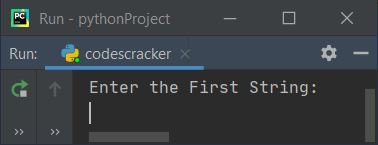Now supply inputs say codes as first and cracker as second string, press `ENTER` key to concatenate given two strings into the third string and print the value of third string as shown in the snapshot given below: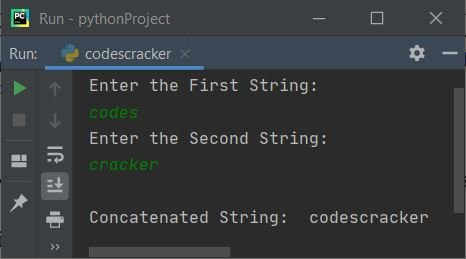#### Modified Version of Previous Program

This program concatenates second string entered by user into the first. The end= is used to skip printing of an automatic newline line through print()

```print("Enter Two Strings: ", end="")
strOne = input()
strTwo = input()

print("\nFirst String before Concatenation: ", strOne)
strOne = strOne + strTwo
print("\nFirst String after Concatenation: ", strOne)```

Here is its sample run with same user input as of previous program's sample run: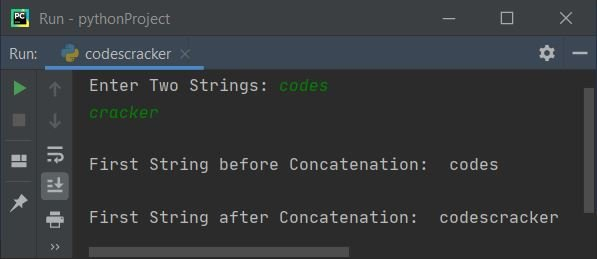## Concatenate String using List

This is the program created through complete user-based code to concatenate two strings. That is, this program uses list to do the job. Let's have a look at the program first:

```print("Enter Two Strings: ", end="")
strOne = input()
strTwo = input()

print("\nFirst String before Concatenation:", strOne)

oneList = []
twoList = []
oneList[:0] = strOne
twoList[:0] = strTwo
oneLen = len(strOne)
twoLen = len(strTwo)

for i in range(twoLen):
oneList.insert(oneLen, twoList[i])
oneLen = oneLen+1

print("\nFirst String after Concatenation: ", end="")
for i in range(len(oneList)):
print(end=oneList[i])
print()```

Here is it sample run with user input codes and cracker as first and second string: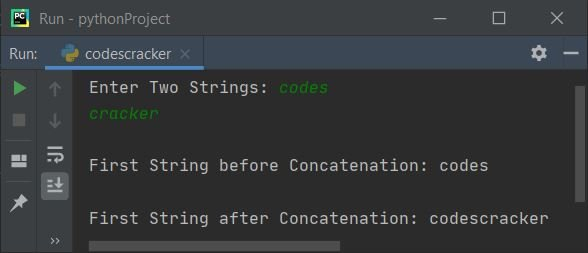The dry run of above program with same user input as given in above sample run, goes like:

• Initial values, strOne=codes and strTwo=cracker, entered by user
• Now using the statement
`oneList = []`
an empty list named oneList is defined
• And using
`oneList[:0] = strOne`
the value of string stored in strOne, that is the first string (codes) gets stored in the list oneList from 0th index in following way:
• oneList = c
• oneList = o
• oneList = d
• oneList = e
• oneList = s
• The statement,
`oneLen = len(strOne)`
is used to find and store the length of strOne to oneLen. So after executing this statement, oneLen = 5. The length of codes
• The range() method returns a sequence of values. It starts with 0 by default, and increments with 1. Stops before the number specified as its argument
• The statement,
`oneList.insert(oneLen, twoList[i])`
is used to insert a character at twoList[i] to the list oneList at its oneLenth index
• That is, at first execution, twoList[i] or twoList or c (very first c of cracker, the second string) gets stored in oneList[oneLen] or oneList
• In this way, one by one, all characters of second string gets stored in first string's list

### Concatenate Both String one by one

This program, concatenates first string to second, and second string to first using list.

```print("Enter Two Strings: ", end="")
strOne = input()
strTwo = input()

oneList = []
twoList = []
tmpList = []
oneList[:0] = strOne
twoList[:0] = strTwo
tmpList[:0] = strOne
oneLen = len(strOne)
twoLen = len(strTwo)
tmpLen = oneLen

for i in range(twoLen):
oneList.insert(oneLen, twoList[i])
oneLen = oneLen+1

print("\nFirst String after Concatenating Second: ", end="")
for i in range(len(oneList)):
print(end=oneList[i])

for i in range(tmpLen):
twoList.insert(twoLen, tmpList[i])
twoLen = twoLen+1

print("\nSecond String after Concatenating First: ", end="")
for i in range(len(twoList)):
print(end=twoList[i])
print()```

Here is its sample run with same user input as of previous program's sample run: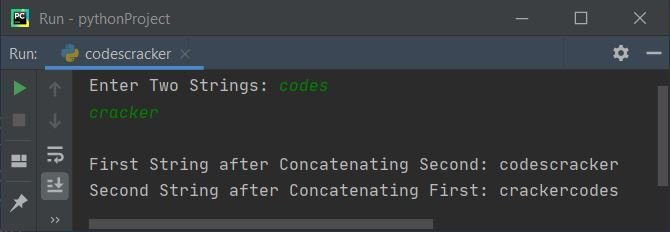Note - We've used third list named tmpList to do the job. That is, this list is used to store the first string's value.

## Concatenate Two Strings using join()

This program uses join() function to join any string to another. Therefore string gets concatenated using join() method as shown in the program given below:

```print("Enter Two Strings: ", end="")
strOne = input()
strTwo = input()

print("\nBefore Concatenation: ", strOne)
strOne = "".join([strOne, strTwo])
print("\nAfter Concatenation: ", strOne)```

Here is its sample run with user input codes and cracker as first and second string: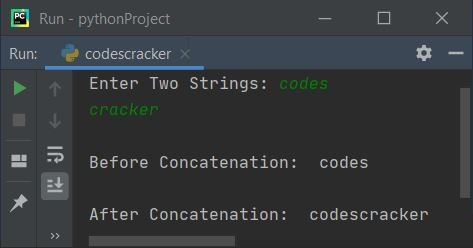To concatenate strings with a single space between them, replace the following statement:

`strOne = "".join([strOne, strTwo])`

with the statement given below:

`strOne = " ".join([strOne, strTwo])`

The sample run is shown in the snapshot given below after modifying this code/statement: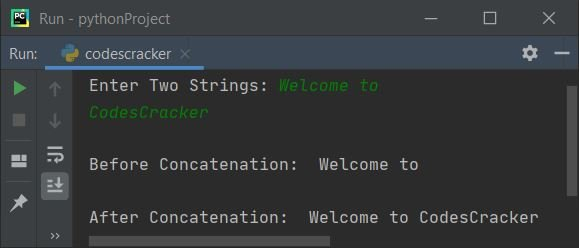#### Same Program in Other Languages

Python Online Test

« Previous Program Next Program »